Classical Approach (Priori Probability), Business Mathematics and Statistics

# Classical Approach (Priori Probability), Business Mathematics and Statistics - Business Mathematics and Statistics - B Com

The origin and development of the theory of probability dates back to the seventeenth century. Ordinarily speaking the probability of an event denotes the likelihood of its happening. A value of the probability is a number ranges between 0 and 1. Different schools of thought have defined the term probability differently.
The various schools of thought which have defined probability are discussed briefly.

Classical Approach (Priori Probability) The classical approach is the oldest method of measuring probabilities and has its origin in gambling games. According to this approach, the probability is the ratio of favourable events to the total number of equally likely events. If we toss a coin we are certain that the head or tail will come up. The probability of the coin coming down is 1, of the head coming up is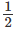and of the tail coming up is. It is customary to describe the probability of one event as 7" (success) and of the other event as ‘q’ (failure) as there is no third event.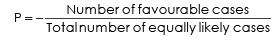If an event can occur in ‘a’ ways and fail to occur in ‘b’ ways and these are equally to occur, then the probability of the event occurring,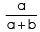is denoted by P. Such probabilities are also known as unitary ortheoretical or mathematical probability. P is the probability of the event happening and q is the probability of its not happening.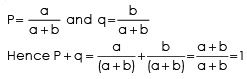Therefore
P + q = 1. 1 – p = q,  1 – q = p Probabilities can be expressed either as ratio, fraction or percentage, such as - or 0.5 or 50%

Limitations of Classical Approach:

1. This definition is confined to the problems of games of chance only and cannot explain the problem other than the games of chance.

2. We cannot apply this method, when the total number of cases cannot be calculated.

3. When the outcomes of a random experiment are not equally likely, this method cannot be applied.

4. It is difficult to subdivide the possible outcome of experiment into mutually exclusive, exhaustive and equally likely in most cases.

Example 1: What is the chance of getting a king in a draw from a pack of 52 cards?
Solution :
The total number of cases that can happen = 52 (52 cards are there).
Total number of kings are 4 ; hence favourable cases=4 Therefore probability of drawing a king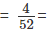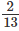Example 2:
Two coins are tossed simultaneously. What is the probability of getting a head and a tail ?
Solution :

The possible combinations of the two coins turning up with head (H) or tail (T) are HH, HT, TH, TT. The favourable ways are two out of these four possible ways and all these are equally likely to happen.
Hence the probability of getting a head and a tail is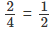Example 3 :
One card is drawn at random from a well-shuffled pack of 52 cards. What is the probability that it will be (a) a diamond (b) a queen ?
Solution :

(a) There are 13 diamond cards in a pack of 52 cards. The number of ways in which a card can be drawn from that pack is 52. The number favourable to the event happening is 13.
Hence probability of drawing a diamond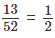(b) There are 4 queens in the pack ; and so the number of ways favourable to the event = 4
The probability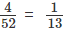Example 4 :
Two cards are drawn from a pack of cards at random. What is the probability that it will be (a) a diamond and a heart (b) a king and a queen (c) two kings ?
Solution :

(a) The number of ways of drawing 2 cards from out of 52 cards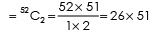The number of ways of drawing a diamond and a heart =13 x 13

The required probability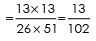(b) The number of ways of drawing a king and a queen = 4 × 4 The required probability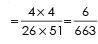(c) Two kings can be drawn out of 4 kings in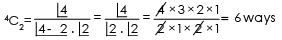The probability of drawing 2 kings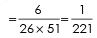Example 5 : A bag contains 7 red, 12 white and 4 green balls. What is the probability that : (a) 3 balls drawn are all white and (b) 3 balls drawn are one of each colour ?
Solution :
(a) Total number of balls = 7 +12 + 4 = 23
Number of possible ways of drawing 3 out of 12 white 12C3
Total number of possible ways of drawing 3 out of 23 balls 23C3

Therefore, probability of drawing 3 white balls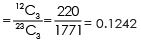(b) Number of possible ways of drawing 1 out of 7 red — 7C1 Number of possible ways of drawing 1 out of 12 white = 12C1 Number of possible ways of drawing 1 out of 4 green - 4C1 Therefore the probability of drawing balls of different   colours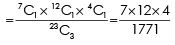= 0.1897

The document Classical Approach (Priori Probability), Business Mathematics and Statistics | Business Mathematics and Statistics - B Com is a part of the B Com Course Business Mathematics and Statistics.
All you need of B Com at this link: B Com

115 videos|142 docs

## FAQs on Classical Approach (Priori Probability), Business Mathematics and Statistics - Business Mathematics and Statistics - B Com

 1. What is the classical approach (a priori probability) in business mathematics and statistics?The classical approach, also known as a priori probability, is a method of determining the probability of an event based on its likelihood in theory or logic. It assumes that all outcomes are equally likely, making it suitable for situations where there is no empirical data available. In business mathematics and statistics, the classical approach is often used as a theoretical foundation for probability calculations.
 2. How is the classical approach applied in business decision-making?The classical approach is commonly applied in business decision-making to assess the probability of various outcomes. By assuming equal likelihood for all possible outcomes, decision-makers can use the classical approach to estimate the likelihood of events occurring. This information is then used to make informed decisions, such as evaluating the feasibility of new projects, estimating market demand, or assessing the risk of certain investments.
 3. What are the limitations of the classical approach in business mathematics and statistics?While the classical approach is a useful tool, it has certain limitations in business mathematics and statistics. One limitation is its reliance on assumptions of equal likelihood, which may not always reflect the real-world probabilities. Additionally, the classical approach does not consider any empirical data or historical trends, which can provide valuable insights in business decision-making. Therefore, it is important to use the classical approach cautiously and complement it with other probability methods when empirical data is available.
 4. How does the classical approach differ from other probability approaches in business mathematics and statistics?The classical approach differs from other probability approaches in business mathematics and statistics primarily in its assumption of equal likelihood for all possible outcomes. This sets it apart from other approaches such as empirical probability, which uses historical data to estimate probabilities, and subjective probability, which relies on personal judgments or expert opinions. The classical approach is often used when there is no empirical data available, or as a theoretical foundation for probability calculations.
 5. Can the classical approach be used in scenarios where outcomes are not equally likely?The classical approach is not suitable for scenarios where outcomes are not equally likely. It assumes that all outcomes have the same probability, which may not be the case in many real-world situations. In such cases, other probability approaches, such as empirical probability or subjective probability, should be used to account for the actual probabilities based on available data or expert opinions. It is important to choose the appropriate probability approach that best reflects the specific scenario at hand.

115 videos|142 docsExplore Courses for B Com examSignup to see your scores go up within 7 days! Learn & Practice with 1000+ FREE Notes, Videos & Tests.
10M+ students study on EduRev
Track your progress, build streaks, highlight & save important lessons and more!
Related Searches

,

,

,

,

,

,

,

,

,

,

,

,

,

,

,

,

,

,

,

,

,

,

,

,

;